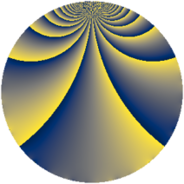Properties

 Label 1728.1.oLevel $1728$ Weight $1$ Character orbit 1728.o Rep. character $\chi_{1728}(127,\cdot)$ Character field $\Q(\zeta_{6})$ Dimension $4$ Newform subspaces $1$ Sturm bound $288$ Trace bound $0$

Related objects

Defining parameters

 Level: $$N$$ $$=$$ $$1728 = 2^{6} \cdot 3^{3}$$ Weight: $$k$$ $$=$$ $$1$$ Character orbit: $$[\chi]$$ $$=$$ 1728.o (of order $$6$$ and degree $$2$$) Character conductor: $$\operatorname{cond}(\chi)$$ $$=$$ $$36$$ Character field: $$\Q(\zeta_{6})$$ Newform subspaces: $$1$$ Sturm bound: $$288$$ Trace bound: $$0$$

Dimensions

The following table gives the dimensions of various subspaces of $$M_{1}(1728, [\chi])$$.

Total New Old
Modular forms 108 8 100
Cusp forms 36 4 32
Eisenstein series 72 4 68

The following table gives the dimensions of subspaces with specified projective image type.

$$D_n$$ $$A_4$$ $$S_4$$ $$A_5$$
Dimension 0 4 0 0

Trace form

 $$4 q + 2 q^{5} + O(q^{10})$$ $$4 q + 2 q^{5} + 2 q^{13} - 2 q^{29} + 2 q^{41} + 2 q^{61} - 2 q^{65} - 2 q^{77} - 2 q^{97} + O(q^{100})$$

Decomposition of $$S_{1}^{\mathrm{new}}(1728, [\chi])$$ into newform subspaces

Label Dim. $$A$$ Field Image CM RM Traces $q$-expansion
$a_{2}$ $a_{3}$ $a_{5}$ $a_{7}$
1728.1.o.a $4$ $0.862$ $$\Q(\zeta_{12})$$ $A_{4}$ None None $$0$$ $$0$$ $$2$$ $$0$$ $$q-\zeta_{12}^{4}q^{5}+\zeta_{12}^{5}q^{7}-\zeta_{12}^{5}q^{11}+\cdots$$

Decomposition of $$S_{1}^{\mathrm{old}}(1728, [\chi])$$ into lower level spaces

$$S_{1}^{\mathrm{old}}(1728, [\chi]) \cong$$ $$S_{1}^{\mathrm{new}}(288, [\chi])$$$$^{\oplus 4}$$$$\oplus$$$$S_{1}^{\mathrm{new}}(576, [\chi])$$$$^{\oplus 2}$$$$\oplus$$$$S_{1}^{\mathrm{new}}(864, [\chi])$$$$^{\oplus 2}$$